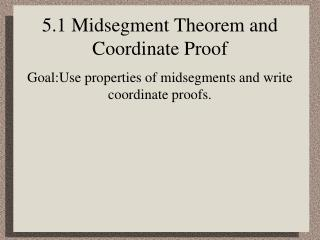Download Presentation5.1 Midsegment Theorem and Coordinate Proof

# 5.1 Midsegment Theorem and Coordinate Proof

Download Presentation## 5.1 Midsegment Theorem and Coordinate Proof

- - - - - - - - - - - - - - - - - - - - - - - - - - - E N D - - - - - - - - - - - - - - - - - - - - - - - - - - -
##### Presentation Transcript

1. 5.1 Midsegment Theorem and Coordinate Proof Goal:Use properties of midsegments and write coordinate proofs.

2. Vocabulary • Midsegment of a triangle:A midsegment of a triangle is a segment that connects the midpoints of two sides of the triangle. • Coordinate proof:A coordinate proof involves placing geometric figures in a coordinate plane.

3. MidsegmentTheorem • The segment connecting the midpoints of two sides of a triangle is parallel to the third side and half as long as that side.

4. Example 1:Use the Midsegment Theorem to find lengths

5. Example 2:Use the Midsegment Theorem

6. Checkpoint

7. http://www.regentsprep.org/Regents/math/geometry/GP10/PracMid.htmhttp://www.regentsprep.org/Regents/math/geometry/GP10/PracMid.htm

8. Example 3:Place a figure in a coordinate plane • Place each figure in a coordinate plane in a way that is convenient for finding side lengths. Assign coordinates to each vertex. • a square b. an acute triangle It is easy to find lengths of horizontal and vertical segments and distances from (0,0), so place one vertex at the origin and one or more sides on an axis.

9. Example 3:Place a figure in a coordinate plane a. Let s represent the side length. The square represents a general square because the coordinates are based only on the definition of a square. If you use this square to prove a result, the result will be true for all squares.

10. Example 3:Place a figure in a coordinate plane b. You need to use three different variables.

11. Example 4:Apply variable coordinates • In Example 3 part (a), find the length and midpoint of a diagonal of the square. • Draw a diagonal and its midpoint. • Assign letters to the points.

12. Checkpoint • Place an obtuse scalene triangle in a coordinate plane that is convenient for finding side lengths. Assign coordinates to each vertex.

13. Checkpoint

14. Summary • You should be able to use the Midsegment Theorem to find lengths in triangles. • You should be able to determine properties of lines in triangles related to midsegments. • You should have an understanding of proving geometric properties using definitions of a geometric figure. • You should be able to use abstract (variable) coordinates to represent geometric figures and relationships.

15. Assignment

16. Things alter for the worse spontaneously, if they be not altered for the better designedly. Francis Bacon